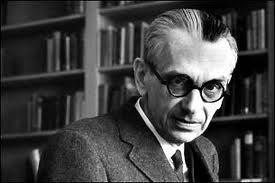• 1906 April 28
(b.) -
1978
(d.)

#### Bio/Description

G?del is best known for his two incompleteness theorems, published in 1931 when he was 25 years of age, one year after finishing his doctorate at the University of Vienna. The more famous incompleteness theorem states that for any self-consistent recursive axiomatic system powerful enough to describe the arithmetic of the natural numbers (Peano arithmetic), there are true propositions about the naturals that cannot be proved from the axioms. To prove this theorem, G?del developed a technique now known as G?del numbering, which codes formal expressions as natural numbers. He also showed that the continuum hypothesis cannot be disproved from the accepted axioms of set theory, if those axioms are consistent. He made important contributions to proof theory by clarifying the connections between classical logic, intuitionistic logic, and modal logic.
• Date of Birth:

1906 April 28
• Date of Death:

1978
• Gender:

Male
• Noted For:

Known for his two incompleteness theorems which heavily influenced the sciences and mathematics
• Category of Achievement: